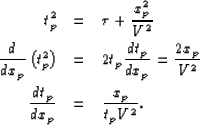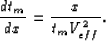Next: REFERENCES Up: Least-squares joint imaging of Previous: HEMNO Equivalence with Levin

# Derivation of Snell Resampling Operator

In the following appendix, I derive the Snell resampling operation, equation (). The graphical basis for the derivation is Figure. Since the pegleg multiple and primary in the figure have the same emergence angle,, the stepout, or spatial derivative, of the traveltime curves of the two events is the same at x and xp. First we compute the stepout of the primary event, starting from the standard NMO equation:(49) (50) (51)
Using equations () and (), we can similarly compute the stepout of the corresponding jth-order pegleg multiple:(52)
Finally, we compute xp as a function of x by squaring equations () and (), setting them equal, and substituting traveltime equations () and () for tm and tp, respectively:(53) (54) (55)Next: REFERENCES Up: Least-squares joint imaging of Previous: HEMNO Equivalence with Levin
Stanford Exploration Project
5/30/2004